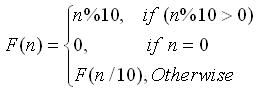favorite We need a little bit of your help to keep things running, click on this banner to learn more
Problems

Let's define a simple recursive function F(n), whereLet's define the function S (p, q) as follows:In this problem you have to calculate S (p, q) on given values of p and q.

#### Input

Consists of multiple test cases. Each line contains two nonnegative integers p and q (pq), separated by space. p and q will fit in 32 bit signed integer. Input is terminated by a line with two negative integers. This line should not be processed.

#### Output

For each pair p and q print the value S (p, q) on a single line.

Time limit 1 second
Memory limit 122.17 MiB
Input example #1
```1 10
10 20
30 40
-1 -1
```
Output example #1
```46
48
52
```